Next: When does relevance feedback Up: Relevance feedback and pseudo Previous: The #rocchio71### algorithm.   Contents   Index

## Probabilistic relevance feedback

Rather than reweighting the query in a vector space, if a user has told us some relevant and nonrelevant documents, then we can proceed to build a . One way of doing this is with a Naive Bayes probabilistic model. If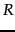is a Boolean indicator variable expressing the relevance of a document, then we can estimate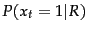, the probability of a term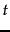appearing in a document, depending on whether it is relevant or not, as: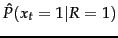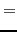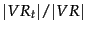(50)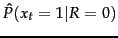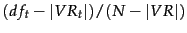(51)

where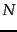is the total number of documents,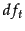is the number that contain,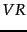is the set of known relevant documents, and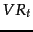is the subset of this set containing. Even though the set of known relevant documents is a perhaps small subset of the true set of relevant documents, if we assume that the set of relevant documents is a small subset of the set of all documents then the estimates given above will be reasonable. This gives a basis for another way of changing the query term weights. We will discuss such probabilistic approaches more in Chapters 11 13 , and in particular outline the application to relevance feedback in Section 11.3.4 (page). For the moment, observe that using just Equation 50 as a basis for term-weighting is likely insufficient. The equations use only collection statistics and information about the term distribution within the documents judged relevant. They preserve no memory of the original query.Next: When does relevance feedback Up: Relevance feedback and pseudo Previous: The #rocchio71### algorithm.   Contents   Index